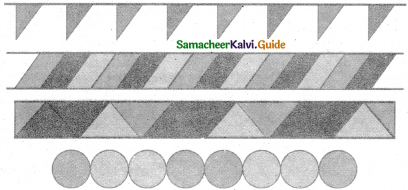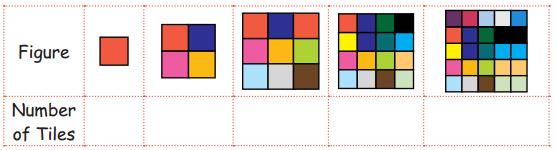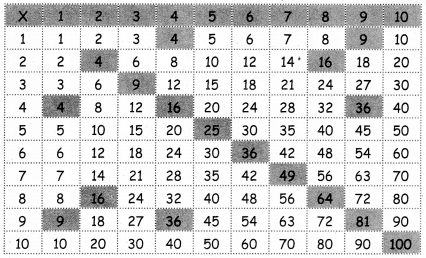Students can download 5th Maths Term 1 Chapter 3 Patterns InText Questions and Answers, Notes, Samacheer Kalvi 5th Maths Guide Pdf helps you to revise the complete Tamilnadu State Board New Syllabus, helps students complete homework assignments and to score high marks in board exams.

## Tamilnadu Samacheer Kalvi 5th Maths Solutions Term 1 Chapter 3 Patterns InText Questions

Activity (Text Book Page No. 53)

Continue the colours pattern as shownDo yourself (Text Book page No. 56)

Question 1.
Count and write the titlesQuestion 2.
Circle the square numbersTry These (Text Book page No. 60)

a. 1 + 3 + 5 + 7 + 9 + 11 = ____ = ____ = ____
b. 1 + 3 + 5 + 7 + 9 + 11 + 13 = ____ = ____ = ____
c. 1 + 3 + 5 + 7 + 9 + 11 + 13 + 15 = ____ = ____ = ____